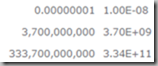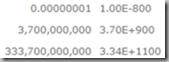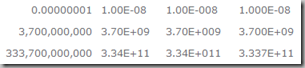## 4/07/2018

### SharePoint TEXT() Function Bug for Scientific Notation

In Excel you can use the TEXT function to display very large and very small numbers in scientific notation.

TEXT([Number],"0.00E+00")Due to a bug in SharePoint Calculated Columns, the TEXT function adds some extra zeros to the end of the formatted number.### Here’s a workaround…

Assuming you want the equivalent of TEXT([Number],"0.00E+00")

```=LEFT(TEXT(Number,"0.00E+00"),6) &
TEXT(MID(TEXT(Number,"0.00E+00"),7,LEN(TEXT(Number,"0.00E+00"))-8),"00")
```

This part:

`LEFT(TEXT(Number,"0.00E+00"),6)`

Pulls off the "3.70E+".

This part:

`MID(TEXT(Number,"0.00E+00"),7,LEN(TEXT(Number,"0.00E+00"))-8)`

Pulls off the "9" and formats it as "09”.

To change the format, adjust the number of zeros in the obvious places, and adjust the "6", "7" and "8" as needed. Here two examples to help you figure out which numbers to change.

For the equivalent of TEXT([Number],"0.00E+000")

```=LEFT(TEXT(Number,"0.00E+000"),6) &
TEXT(MID(TEXT(Number,"0.00E+000"),7,LEN(TEXT(Number,"0.00E+000"))-9),"000")```

For the equivalent of TEXT([Number],"0.000E+00")

```=LEFT(TEXT(Number,"0.000E+00"),7) &
TEXT(MID(TEXT(Number,"0.000E+00"),8,LEN(TEXT(Number,"0.000E+00"))-9),"00")
```

Here the "0.00E+00", "0.00E+000" and "0.000E+00" results.### Added to sharepoint.uservoice.com

I just listed this as a bug. If you would like to vote for a fix, go here: https://sharepoint.uservoice.com/forums/329214-sites-and-collaboration/suggestions/33878629-fix-text-function-bug-for-scientific-notation

As the feature has minimal usage, and has been there forever, I don't expect too much... ;-)

.

## Note to spammers!

Spammers, don't waste your time... all posts are moderated. If your comment includes unrelated links, is advertising, or just pure spam, it will never be seen.